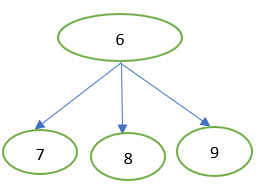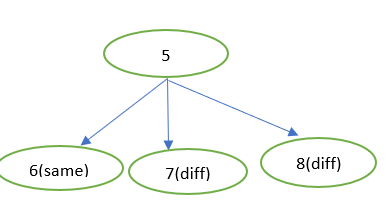# A Good Problem - Approach and Doubts

Recently I had participated in a contest on Hackerearth and came across this problem : Numbers Game

Problem Statement Summary

This is a game between two persons : Robert and Ned .
There are two numbers N and K provided to us.
Robert Starts and picks a number , say x between 1 and K
Ned then picks a number (let’s call the number y) between x+1 and x+K
Now Robert’s turn again and he picks a number between y+1 and y+K and so on and so forth.
The first person to reach N wins. Both persons play optimally
We must determine who will WIN this game !!

Sample Case Explanation

If N = 3 and K = 3 ,
Robert plays first and can choose a number between 1 and K , here he can choose 3 which is in fact N and ends up winning the game !

If N = 4 and K = 1 ,
Robert’s Turn : Has to pick between 1 and K , in this case , he can pick only 1 , Let us call this choice_1 = 1
Ned’s Turn : Has to pick between choice_1+1 and choice_1+K => In this case , 2 so
choice_2 = 2
Robert’s Turn : Has to pick between choice_2+1 and \$choice_2+K =>\$ 3 so choice_3 = 3
Ned’s Turn : By Similar Reasoning , choice_4 = 4 , which is actually N and game ends here making Ned the winnerTry to think a bit on how you would approach it before moving forward !

My Approach , Thoughts and Observations

Initially of course , my head was pretty muddled up because this was a short contest requiring you to find the solution really quick and I did not know how to simulate the players playing optimally

I was stuck.

Then I started thinking of trivial cases , if K >= N , obviously in the first turn itself when Robert plays and has to select a number between 1 and K , he can select N and conclusively win there and then

Then I was fumbling a bit with the idea of N-K as I thought if a player got to / chose N-K in any move , the player in the next move will win as he can select N in the next move .

The third thing that caught my attention was the low constraints on N and K , both were under 1001 indicating that an O(N^2) solution would pass.

Now a solution started forming in my mind :
Let’s look at the numbers from back to front i.e. from N to 1 , I took the example of N=10 and K=3 so we look at the sequence of numbers

Let’s begin :

If some player is at the number 10 , He is the WINNER so the same person who obtains 10 is the winner . Next

If a player is at 9 or is forced to choose 9 , then the same player cannot win because obviously in the next turn the other player will choose 10 which is N as the next player is allowed to choose from 9+1 to 9+K => 10 to 13 so different or other player will win . Next

Say a player chooses 8 , then the next player who plays after him can choose between 8+1 and 8+K which is 9 to 11 range , he chooses 10 obv so next or different player wins . Next

If a player is at 7 , the next player after him can choose from 8 to 10 , so he chooses 10 and different or next player wins . Then

Now the real fun begins , if a player is at 6 , the next player can choose from 7 to 9 (From 6+1 to 6+K )Next player can choose b/w 7 to 9
But we already know that if a player arrives at 7 , the other player will win
If a player arrives at 8 , the next / other / different player will win
If a player arrives or chooses 9 , other or next player will win

So if a player chooses 6 as we saw in the root node , no matter what the next player chooses , the same or original player , the one who chose 6 will WIN . Why ?
Let’s say Player A chooses 6
Player B chooses 7 then next turn Player A chooses 10
Player B chooses 8 then next turn Player A chooses 10
Player B chooses 9 then next turn Player A chooses 10
No matter what happens the original player who chose 6 (Be It Player A or B ) will WIN .
Next …

If some player chooses 5 will we lose/win ?
Let’s look at the choices the other player hasWe know that if a player chooses 6 that player will win => same player
If a player chooses 7 , the other player will win
If a player chooses 8 , other player will win .

Now both of these players play optimally so if some player chooses 5 , the next player will look the options , 6 , 7 and 8 , he sees that if he chooses 6 , he wins so he takes that therefore the player who chooses 5 DOES NOT WIN i.e. the different player than the one who chose 5 wins .
Scenarios to prove
Player A chooses 5
Player B chooses 6
If Player A chooses 7 , 8 or 9 , in the next turn Player B can choose 10 and win .
Similar case when Player B chooses 5 , Player A chooses 6 , whatever Player B chooses , next turn Player A will win by choosing 10
The Player who chooses 5 DOES NOT WIN , the different or other player wins.

Similarly try by yourself to determine , if a player chooses 4 , does that same player win or different ?
and for 3 , 2 and 1You will get this “result” array for indices 10 to 1 :I denote 0 as same and 1 as different , as we’ve seen if a player gets to choose 10 , he wins so result = 0
Similarly result , result , result = 1 as the other player and not the player who chooses 7 , 8 , 9 wins and so on . Again the array is printed from index 10 to index 1

I just loop through this array from index 1 to K which is what Rob starts out with , if at least one SAME is present Rob wins else Ned wins i.e. if count of 0 in result array from indices 1 to K is greater than equal to 1 , then Rob WINS .
Why ? Because , if one of the value is same , then the guy who chooses that number , the same guy wins by our reasoning above !

Code

``````#include <bits/stdc++.h>
using namespace std ;
int main()
{

//0 denotes same and 1 denotes opposite
int t;
cin >> t ;
while(t--)
{

long long int n , k ;
int result[20000+1] ;
cin >> n >> k ;
//trivial case ?
if(k>=n)
cout << 0 << endl ;
else{
int same = 0 ;
for(int i=n ; i>=1 ; i--)
{
if(i==n)
result[i] = 0 ;
else if(i>=n-k && i<n)
result[i] = 1 ;
else
{
same = 0 ; //count of same
for(int sec = i+1 ; sec<=i+k ; sec++)
{

if(sec<=n && result[sec]==0)
same++ ;
}
if(same>=1)
result[i] = 1 ;
else
result[i] = 0;

}
}
same = 0 ;
for(int i=1 ; i<=k ; ++i)
{
if(i<=n && result[i]==0)
same++ ;
}
if(same>=1)
cout << 0 << endl;
else
cout << 1 << endl;
}
}
return 0 ;
}

``````

And I got AC , I was so happy !!!
The question states to output 0 if Rob wins else 1 . The code above was the one I used in contest so it is not well documented and might be obfuscated . Let me know if I need to re-write a clean version will heavy comments if you don’t understandMy Detailed editorial on MSV

Doubts

1. Is my approach correct . I’ve read a lot of @tmwilliamlin’s comments about upsolving and trying questions that make you struggle and proving solutions and this question did make me struggle a bit and I was pretty happy instead of a pattern , I can justify why the solution worked
2. Is there a faster way to solve this problem and how
3. I know practise is the key to solving questions like these faster but what’s the most effective way to do guided practise on codechef

I’ve seen amazing coders like @ashishgup participate in this contest , it would be nice if they share their approachesI’ve said it before that @viju123 and @ssjgz and now @tmwilliamlin are the best at this editorial business but I like to try my luck from time to time !

Let me know how it was .

• Enjoyed it ! Do more of these• Hated It

0 voters

Cya Later!!

6 Likes

Ya. I don’t remember the name, but there are certain power(?) States, which are normally sparse. In these states player 2 wins. As long as you can get your opponent to such a state, you can win over there, else you can’t. You essentially just simulated that reasoning. And your approach is completely correct.
A lot of turn based game theory can be solved by applying it. Even in harder questions, You can easily use it to generate the answers for small cases to allow easier observation.

1 Like

Interesting , Thank YouI’d better start solving game theory problems soon !
In your opinion , is there a way to solve the problem faster or by a different approach ?

1 Like

I think if can be done in O(1) but the constraints are low, so I’m not sure, give me some time.

``````#include<iostream>
using namespace std;
int main(){
int t;
cin>>t;
while(t--){
int n,k;
bool ans;
cin>>n>>k;
if(n%(k+1)){
ans=0;
}
else{
ans=1;
}
cout<<ans<<'\n';
}
}
``````

Yup, it can.

3 Likes

Same problem from HackerEarth June Circuits, just the language is different

1 Like

That’s really cool xD
I thought of something with mod initially ;(
How did you come up with it tho? Could you explain the reasoning .
Compared to my logic, this is amazing.
The only times I can get solutions like these is by observing a lot of example cases.

Yeah, got itIt was pretty simpleI un-necessarily complicated it